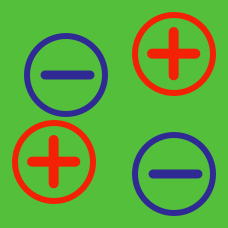Electricity and Magnetism

# Problem solving - charges and fields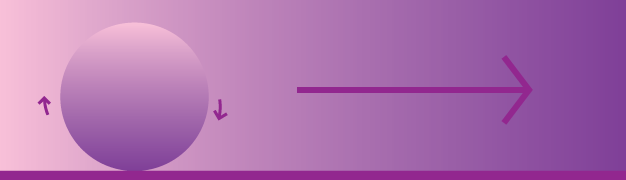A homogeneous ball with mass $m=1 ~\mbox{g}$ and total charge $q=10^{-3}~\mbox{C}$ (uniformly distributed) is placed on a horizontal $xy$-plane. The ball starts rolling without slipping under the influence of a uniform electrostatic field $\vec{E}=(E,0,0)$ with $E=1~ \mbox{V/m}.$ Find the acceleration in meters per second squared of the center of mass of the ball.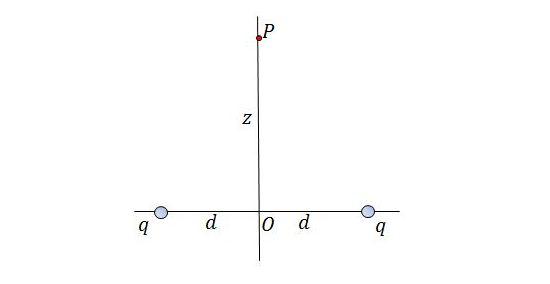If a point charge $Q = -3 \text{ C }$ with mass $m = 1 \text{ kg}$ is put at point $P$ which is at a distance of $z = 9 \text{ m}$ above the midpoint between two equal charges $q = 25 \times 10^{-12} \text{ C },$ at a distance of $2d =24 \text{ m}$ apart, the point charge will be move to point $O.$ If the speed of the point charge at point $O$ can be expressed as $v = \sqrt{\frac{a}{b}} \text{ m/s},$ where $a$ and $b$ are coprime positive integers, what is the value of $a+b?$

Let $\pi \approx 3,$ and the permitivity of free space $\epsilon_0 \approx 9\times 10^{-12} \frac{\text{C}^2}{\text{N*m}^2}.$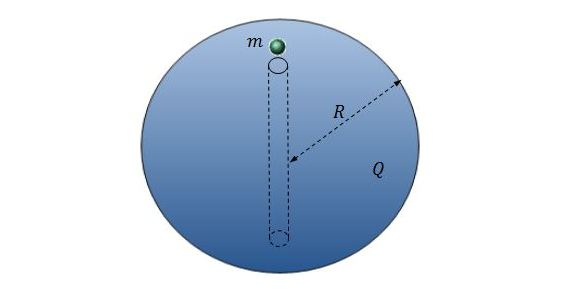There is a point charge with mass $m = 1 \text{ kg}$ and charge $q = -9\times 10^{-6} \text{ C }$ on a uniformly charged sphere with charge $Q = 9\times 10^{-6} \text{ C }$ and radius $R = 1 \text{ m}$. As shown in the above diagram, there is a hole on the sphere, so the point charge can move into the hole. If the period of the point charge can be expressed as $T = 2\pi \sqrt{\frac{a}{b}} \text{ s},$ where $a$ and $b$ are coprime positive integers, what is the value of $a+b?$

Let $\pi \approx 3,$ and the permitivity of free space $\epsilon_0 \approx 9\times 10^{-12} \frac{\text{C}^2}{\text{Nm}^2}.$

A point particle with mass $m = 1 \text{ kg}$ and charge $q = -7\times 10^{-6} \text{ C }$ is rotating around another point particle with charge $Q = 7\times 10^{-6} \text{ C }$,which is fixed at the center, with radius $r = 2 \text{ m}$. If the speed of the circular motion can be expressed as $v = \sqrt{\frac{a}{b}} \text{ m/s},$ where $a$ and $b$ are coprime positive integers, what is the value of $a+b?$

Let $\pi \approx 3,$ and the permitivity of free space $\epsilon_0 \approx 9\times 10^{-12} \frac{\text{C}^2}{\text{N*m}^2}.$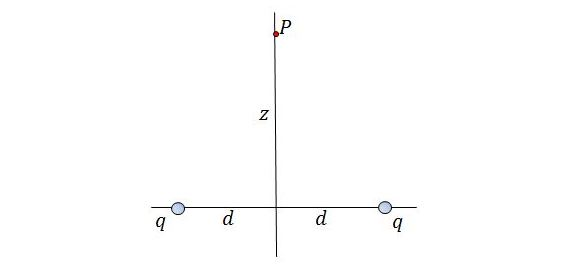The potential at point $P$ which is at a distance of $z = 6 \text{ m}$ above the midpoint between two equal charges $q = 500 \times 10^{-12} \text{ C }$, at a distance of $2d =16 \text{ m}$ apart, can be expressed as $U = \frac{a}{b} \text{ J/C},$ where $a$ and $b$ are coprime positive integers. What is the value of $a+b?$

Let $\pi \approx 3,$ and the permitivity of free space $\epsilon_0 \approx 9\times 10^{-12} \frac{\text{C}^2}{\text{N*m}^2}.$

×